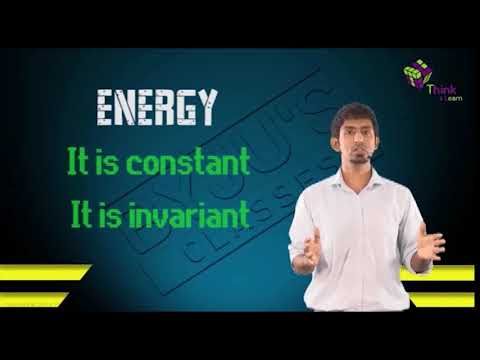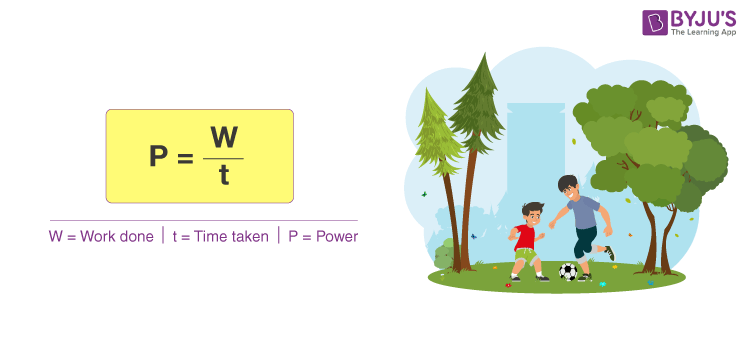# Work, Energy and Power

Work, energy and power are the most used terms in Physics. They are probably the first thing you learn in your Physics class. Work and energy can be considered as two sides of the same coin. In this article, we will learn all about the concept of work, power and energy.

Work done is generally referred in relation to the force applied while energy is used in reference to other factors such as heat. Power is defined as work done per unit time.

## What is Work, Energy and Power?

### Work

Definition Work is said to be done when a force applied to an object moves that object.
Formula We can calculate work by multiplying the force by the movement of the object.

W = F × d

Unit The SI unit of work is the joule (J)

### Energy

Definition In physics, we can define energy as the capacity to do work.
Formula For the potential energy the formula is

P.E. = mgh

Unit The SI unit of energy is joules (J), which is named in honour of James Prescott Joule.

### Power

Definition Power can be defined as the rate at which work is done i.e. energy converted.
Formula The formula for power is

P = W/t

Unit The unit of power is watt (W).## What is Work?

Work is said to be done when a body or object moves with the application of external force. We can define work as an activity involving a movement and force in the direction of the force. For example, a force of 30 newtons (N) pushing an object 3 meters in the same direction of the force will do 90 joules (J) of work.

### Formula of Work

When we kick a football, we are exerting an external force called F and due to this force (kick), the ball moves to a certain distance. This disposition of ball from position A to B is known as displacement (d). This work is said to be done and can be calculated as W = F × d

 Work = Force × Displacement = F × d

### Unit of Work

If a force of 5 newtons is applied to an object and it moves 2 meters, the work will be 10 newton-meter. Newton meter in termed and Joules and it is the unit of Work.

### Example of work

An object is horizontally dragged across the surface by a 100 N force acting parallel to the surface. Find out the amount of work done by the force in moving the object through a distance of 8 m

We know that,

F = 100 N

d = 8 m

Since F and d are in the same direction,

θ = 0, [θ is the angle of the force to the direction of movement]

W = F Cos θ

= 100 x 8 x Cos 0

= 800 J [Since Cos 0 = 1]

## What is Energy?

Energy is the ability to perform work. Energy can neither be created nor destroyed. It can only be transformed from one kind to another. The unit of Energy is same as of Work i.e. Joules. Energy is found in many things and thus there are different types of energy.

All forms of energy are either kinetic or potential. The energy in motion is known as Kinetic Energy whereas Potential Energy is the energy stored in an object and is measured by the amount of work done.

### Types of energy

Some other types of energy are given below:

• Mechanical energy
• Mechanical wave energy
• Chemical energy
• Electric energy
• Magnetic energy
• Nuclear energy
• Ionization energy
• Elastic energy
• Gravitational energy
• Thermal energy
• Heat Energy

### Unit of energy

The SI unit of energy is joules (J), which is named in honour of James Prescott Joule.

## What is Power?

Power is a physical concept that has several different meanings, depending on the context and the information that is available. We can define power is the rate of doing work. It is the amount of energy consumed per unit time.

### Formula of power

As discussed power is the rate of doing work. Therefore, it can be calculated by dividing work done by time. The formula for power is given below.

 $P = \frac{W}{t}$

Where,

P = Power

W = Work done

T = Time taken

### Unit of Power

As power doesn’t have any direction, it is a scalar quantity. The SI unit of power is Joules per Second (J/s), which is termed as Watt. Watt can be defined as the power taken to do one joule of work in one second. The unit Watt is dedicated in honour of So James Watt, the developer of the steam engine.

Click on the link provided below to learn the SI unit of power in detail.

### Example of Power

A garage hoist lifts a truck up 2 meters above the ground in 15 seconds. Find the power delivered to the truck. [Given: 1000 kg as the mass of the truck]

First we need to calculate the work done, which requires the force necessary to lift the truck against gravity:

F = mg = 1000 x 9.81 = 9810 N.

W = Fd = 9810N x 2m = 19620 Nm = 19620 J.

The power is P = W/t = 19620J / 15s = 1308 J/s = 1308 W. P=f.v

### Work, Power and Energy Questions

1. What is the relationship of work energy and power?
2. What happens to the energy as work is done?
3. What is the difference between work energy and power?
4. Is energy transferred the same as work done?
5. How does work affect an object’s energy?
6. How is work energy and power related to each other?
7. How are force energy and work related?
8. What is the formula of work energy and power?
9. How do you calculate energy from power?
10. Can force be converted into energy?

### Important Resources for Work, Energy and PowerThus we have discussed three elementary topics on Physics as students would have covered them during their high school days. We at BYJU’S are of the opinion that proper guidance with the right textbooks is essential for students to succeed. Stay tuned with BYJU’S to learn more about other related physics topics.

1. Tumininu

Lovely

1. Nikitha

Its so useful love this byju’s

1. 👍

2. No need

Very good explanation

3. aliza ansari

thanks

4. nice

5. Meghashyam

Good

Good

6. Tejasvi Chourasia

Excellent explaination done
5 stars to BYJU’S

7. vaibhav

it is very nice because it become easy.

8. Emmanuel lomude

Enjoyable

9. Very nice

10. Lakshay Panwar

very nicely done

11. mutund kalwiji

well explained

12. sanjay singh

really good platform to learn basic

13. Tenzin Zomkit

Thanks a lot . It was very helpful . Lots of love for all your team .

14. Samuel

I really love this explanation

Thank you, this explanation is very useful for this topic.

16. Netra Pareek

Amazing! Today was my online class test, the content given here helped me a lot. I would like to give BYJU’S five stars.

17. MANPREET

Good explanation 👍👍👍👍👍👍

18. Really good explanation
It was very useful
Thank you so much